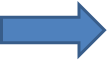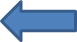BBCNOUN NOTICE BOARDATTENTION: PLEASE NOTE THAT THIS IS NOT THE OFFICIAL NOUN WEBSITE, THE OFFICIAL NOUN WEBSITE REMAINS NOU.EDU.NG

CLICK HERE to contact BBCNOUN on whatsappto Join BBCNOUN Whatsapp group

BREAKING NEWS: CARRYOVER REGISTRATION NOW ON!.

Returning students: Returning students can now register on the portal for second semester, CLICK HERE now.

LOAD WALLET: GENERATE REMITA CODE (RRR) AND LOAD WALLET, CLICK HERE now.

BBCNOUN
(FOUNDER)Registration Date: 06-03-2019
Date of Birth: May 19
Local Time: 08-19-2019 at 08:59 PM
Status: OfflineBBCNOUN's Forum Info
Registration Date:
06-03-2019
Last Visit:
08-17-2019, 10:57 AM 10:57 AM
Total Posts:
99 (1.27 posts per day | 84.62 percent of total posts)
Total Threads:
93 (1.2 threads per day | 93 percent of total threads)
Time Spent Online:
6 Days, 9 Hours, 16 Minutes
Members Referred:
6
Total Likes Received: 1 (0.01 per day | 50 percent of total 2)
Total Likes Given: 1 (0.01 per day | 50 percent of total 2)
Reputation:
BBCNOUN's Contact Details

Additional Info About BBCNOUN
Location:
Lagos, Nigeria
Sex:
Male
BBCNOUN's Signature
Watch and Pray!BBCNOUN's Most Liked Post
Post Subject Numbers of Likes
REGISTRABLE COURSES & COST ESTIMATE FOR COMPUTER SCIENCE 100L - 400L 1
 Thread Subject Forum Name REGISTRABLE COURSES & COST ESTIMATE FOR COMPUTER SCIENCE 100L - 400L ALL NOUN DISCUSSIONS
Post Message
COMPUTER SCIENCE COURSE OUTLINE

100L 1ST SEMESTER
GST101=2,000
GST107=2,000
BIO101= 2,000
CHM103=2,000
CIT101= 2,000
CIT143=2,000
PHY101=2,000
BIO191=2,000
CHM191=2,000
PHY191=2,000
CHM101=2,000
MTH101=2,500
MTH103 =2,500
COMPULSORY FEE=36,000
COURSE REG FEE=27,500
EXAM REG FEE=13,000
SCHOOL FEE=76,500

100L 2ND SEMESTER
GST102=2,000
CHM102=2,000
CIT102=2,000
STT102=2,000
MTH102=2,000
BIO102=2,000
BIO192=2,000
CHM192=2,000
PHY102=2,500
PHY192=2,000
CIT104=2,000
COMPULSORY FEE=18,000
COURSE REG FEE=22,500
EXAM REG FEE=11,000
SCHOOL FEE=51,500

200L FIRST SEMESTER
GST201=2,000
GST203=2,000
CIT211=2,500
CIT213=2,000
CIT215=2,500
CIT237=2,500
MTH213=2,500
MTH281=2,500
COMPULSORY FEE=18,000
COURSE REG FEE=18,500
EXAM REG FEE=8,000
SCHOOL FEE=44,500

200L 2ND SEMESTER
GST202=2,000
CIT208=2,000
CIT212=2,500
CIT246=2,000
CIT292=2,000
MTH212=2,500
MTH282=2,500
COMPULSORY FEE=18,000
COURSE REG FEE=15,500
EXAM REG FEE=7,000
SCHOOL FEE=40,500

300L 1ST SEMESTER
CIT309=2,500
CIT311=2,500
CIT333=2,500
CIT341=2,500
CIT351=2,000
CIT353=2,500
CIT371=2,500
CIT383=2,000
COMPULSORY FEE=18,000
COURSE REG FEE=18,500
EXAM REG FEE=8,000
SCHOOL FEE=44,500

300L 2ND SEMESTER
CIT322=2,500 C
CIT342=2,500 C
CIT344=2,500 C
CIT381=2,000 C
CIT389=10,000 C- I.T/SIWES
CIT392=2,000 C
MTH382=2,500 E
COMPULSORY FEE=18,000
COURSE REG FEE=24,000
EXAM REG FEE=6,000
SCHOOL FEE=48,000

400L 1ST SEMESTER
CIT403=2,500 C
CIT411=2,000 C
CIT425=2,500 C
CIT427=2,500 C
CIT445=2,500 C
CIT465=2,500 E
COMPULSORY FEE=18,000
COURSE REG FEE=14,000
EXAM REG FEE=6,000
SCHOO FEE=38,000

400L 2ND SEMESTER
CIT412=2,500 C
CIT432=2,500 C
CIT474=2,000 E
CIT484=2,000 C
CIT499=15,000 C (PROJECT)
COMPULSORY FEE=18,000
COURSE REG FEE=24,000
EXAM REG FEE=4,000
SCHOOL FEE=36,000